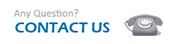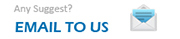JDK 源码解析 — Integer

1. public static Integer valueOf(int i) {
2.     assert IntegerCache.high >= 127;
3.     if (i >= IntegerCache.low && i <= IntegerCache.high)
4.         return IntegerCache.cache[i + (-IntegerCache.low)];
5.     return new Integer(i);
6. }

1. private static class IntegerCache {
2.     static final int low = -128;
3.     static final int high;
4.     static final Integer cache[];
5.
6.     static {
7.         // high value may be configured by property
8.         int h = 127;
9.         String integerCacheHighPropValue =
10.             sun.misc.VM.getSavedProperty("java.lang.Integer.IntegerCache.high");
11.         if (integerCacheHighPropValue != null) {
12.             int i = parseInt(integerCacheHighPropValue);
13.             i = Math.max(i, 127);
14.             // Maximum array size is Integer.MAX_VALUE
15.             h = Math.min(i, Integer.MAX_VALUE - (-low) -1);
16.         }
17.         high = h;
18.
19.         cache = new Integer[(high - low) + 1];
20.         int j = low;
21.         for(int k = 0; k < cache.length; k++)
22.             cache[k] = new Integer(j++);
23.     }
24.
25.     private IntegerCache() {}
26. }

static 静态代码块可知缓存的初始化是在第一次使用的时候。 通过 VM 参数 -XX:AutoBoxCacheMax=<size> 可以配置缓存的最大值。 在 VM 初始化期间， 缓存最大值 high， 可能被保存在 sun.misc.VM class 的私有系统属性里。